# Queueing theory

(diff) ← Older revision | Latest revision (diff) | Newer revision → (diff)

The branch of probability theory in which one studies mathematical models of various kinds of real queues (cf. Queue). These models are presented as random processes of a special form, sometimes called service processes. The definitions of these processes are most often descriptive, since their formal construction is very complicated and not always effective.

Queueing theory mainly uses the apparatus of probability theory. The fundamental problems of queueing theory usually are these: Based on "local" properties of the random processes under discussion, study their stationary characteristics (if they exist) or the behaviour of these characteristics over a long period of time. One of the main aims of research in this area is the choice of a preferable organization for a queueing system.

For example, for a typical object of queueing theory such as an automatic telephone exchange (see Queue with refusals) one of the basic characteristics is the proportion of calls lost, that is, the limit, as(if it exists), of the ratios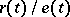of the number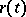of calls lost up to timeto the numberof calls which arrived up to the same time. Here the distribution of the time intervals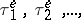between the arrivals of calls and the distribution of the service times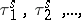of these calls are assumed to be known. The assignment of the distribution of a random (control) sequence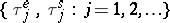together with the description of an algorithm of how the queue evolves form the initial data, characterizing the "local" properties of the service process.

Similarly, for multi-server queues (cf. Queue, multi-channel with waiting) one studies the limit asof the distributions of the probabilitiesandfor the time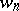which the-th call arriving in the system has to wait before the beginning of its service and the queue lengthat the time of its arrival. The limit distribution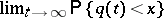for the queue length at time, etc., are also discussed. Here, again, the initial data are the assignment of the control sequences (the distribution of the intervals of service times) and the algorithm defining the operation of the queue.

For relatively simple queues, and under certain assumptions on the control sequence, the required characteristics can be found by analytic methods. However, the number of such systems is not large. The nature of the conditions imposed on the control sequence can be demonstrated by the example of a queue with refusals (an automatic telephone exchange). Let 1) the random variables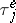be exponentially distributed:that is, the input stream is Poisson; 2) the variablesbe independent and identically distributed and not dependent on. Then the probability of refusal, defined above, exists and is equal to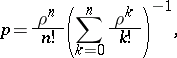whereis the ratio of the mathematical expectations:For this system, if one of the conditions 1) or 2) fails, then the search of explicit formulas forbecomes very complicated or even impossible.

Underlying the analytic approach to the search for explicit expressions for the desired characteristics is a method connected with the construction of Markov processes describing the state of the system. These processes are very well studied and the solution of the problem in this case reduces to the formulation and solution of the corresponding equations for stationary distributions (invariant measures).

Such a process is often used in a modified form by forming semi-Markov processes or imbedded Markov processes (the Markov property is satisfied only at certain random times).

For certain complicated queues exact analytic methods, as a rule, are not effective and one uses asymptotic methods or simulates the random processes by a Monte-Carlo method. Asymptotic methods are relevant in those cases when the system under consideration is close (in its local properties) to another system which is either well studied and allows the calculation of the necessary characteristics, or is in some sense critical. The direction of asymptotic investigation in the first case is described by stability (or continuity) theorems. In the second case stationary characteristics often do not exist, but one can establish "collective" limit theorems for them, that is, theorems in which the limit behaviour is determined not by individual properties of the control sequences but only by certain numerical parameters. Examples of the second type are theorems on the so-called single-server queues with heavy traffic. They have been established as follows. Let the inter-arrival intervals,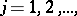and the inter-service times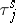,form mutually-independent sequences of independent identically-distributed variables and let the expectation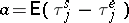of the difference betweenandbe positive. Then there is a non-degenerate limit distributionfor the waiting timeof the-th call. In addition, let the random variables,vary so that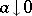(tends to zero from the positive side), so that the variance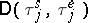converges to a positive limit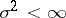and so that the expectationis uniformly bounded for some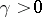. Then for each fixed,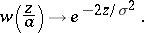(*)

This relation allows the approximate calculation offor small values of. The limit system, corresponding to, is critical in the sense that for it there is no non-degenerate limit distribution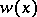, andfor each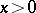(the waiting time of the-th client increases unboundedly asincreases). Relation (*) can be extended to a broad class of queues with waiting under very general assumptions on the control sequences.

The emergence of queueing theory has produced an interest in the novel mathematical problems which arise in the organization of telephone networks. The first publication in this connection is due to A. Erlang in 1907. The later development of queueing theory occurred in the 1940's and 1950's in papers by C. Palm, F. Pollaczek, A.Ya. Khinchin, and others. The last coined the term "queue" . In the USSR, work on queueing theory was continued by B.V. Gnedenko, with a group of his students, and others.

The development of queueing theory has been stimulated both by a variety of applications and by the mathematical content of the problems that arise. Although formally a part of the theory of random processes, queueing theory has evolved into an independent area of research with its own problems and methods of solution.

How to Cite This Entry:
Queueing theory. Encyclopedia of Mathematics. URL: http://encyclopediaofmath.org/index.php?title=Queueing_theory&oldid=18989
This article was adapted from an original article by A.A. Borovkov (originator), which appeared in Encyclopedia of Mathematics - ISBN 1402006098. See original article